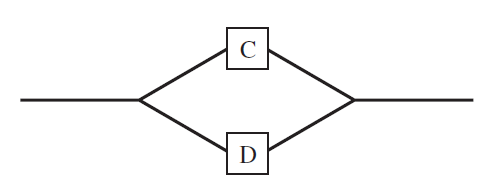×
Get Full Access to Statistics For Engineers And Scientists - 4 Edition - Chapter 2.3 - Problem 37e
Get Full Access to Statistics For Engineers And Scientists - 4 Edition - Chapter 2.3 - Problem 37e

×

# A system contains two components, C and D, connected inISBN: 9780073401331 38

## Solution for problem 37E Chapter 2.3

Statistics for Engineers and Scientists | 4th Edition

• Textbook Solutions
• 2901 Step-by-step solutions solved by professors and subject experts
• Get 24/7 help from StudySoup virtual teaching assistantsStatistics for Engineers and Scientists | 4th Edition

4 5 1 335 Reviews
19
0
Problem 37E

A system contains two components, C and D, connected in parallel as shown in the diagram.Assume C and D function independently. For the system

to function, either C or D must function.

a. If the probability that C fails is 0.08 and the probability that D fails is 0.12, find the probability that the system functions.

b. If both C and D have probabilityof failing, what must the value ofbe so that the probability that the system functions is 0.99?

c. If three components are connected in parallel, function independently, and each has probabilityof failing, what must the value ofbe so that the probability that the system functions is 0.99?

d. If components function independently, and each component has probability 0.5 of failing, what is the minimum number of components that must be connected in parallel so that the probability that the system functions is at least 0.99?

Step-by-Step Solution:

Step 1 of 4

a) Here C and D are 2 conductors connected parallely  and function independently

P(C fails)=P(C’)

=0.08

P(C function)=P(C)

=1-0.08

=0.92

P(D fails)=P(D’)

=0.12

P(D function)=P(D)

=1-0.12

=0.88

P(That the system function)=P(CUD)

=P(C)+P(D)-P(C)P(D)

=0.92+0.88-0.92(0.88)

=0.9904

Step 2 of 4

Step 3 of 4

##### ISBN: 9780073401331

Unlock Textbook Solution